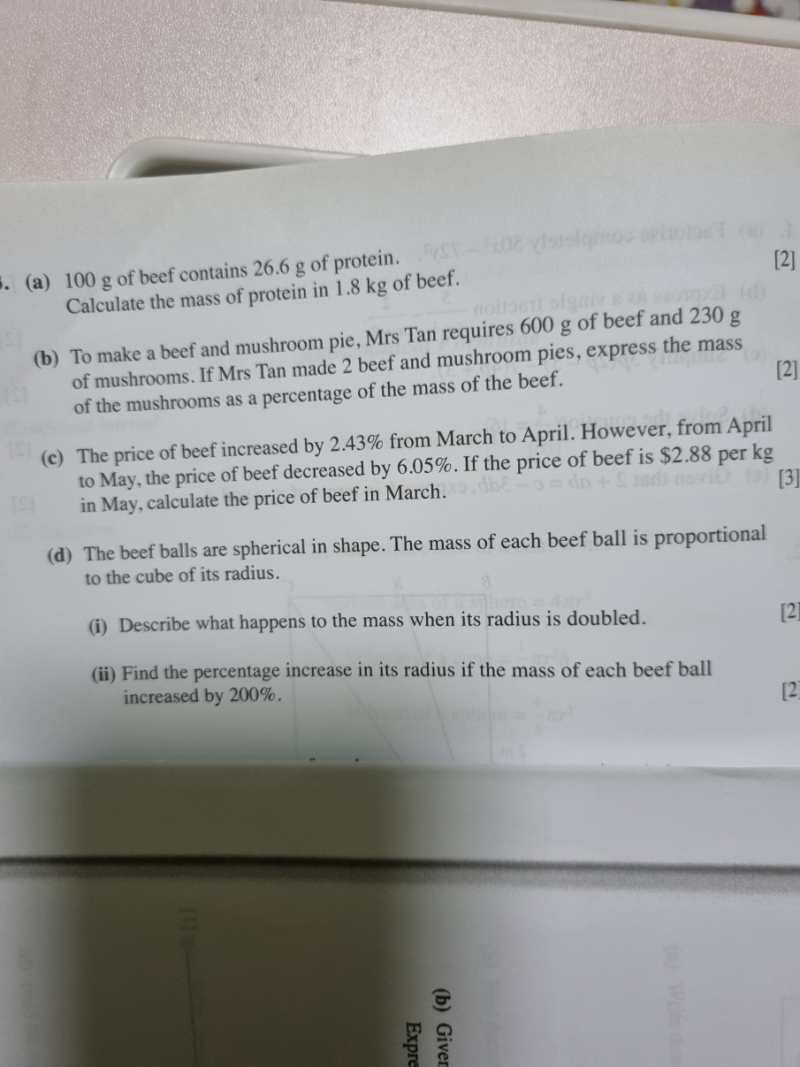# QuestionConfused.pls help.thank you.

(a)  100 g –> 26.6

1800g –> 26.6/100  x 1800 = 478.8

Hence 1.8kg beef has 0.4788 kg protein

(b)                     Beef                                 Mushroom

1 pie            600                                      230

2 pies          600x 2 = 1200             230 x 2 = 460

460 / 1200 x 100 =   38⅓ %

% of mass of mushrooms/ beef  is   38⅓ %

(c)  May price —-> \$2.88

93.95%  —>  \$2.88

100%     —-> \$3.07   = price in April

102.43% —> \$3.07

100%   —–> \$3.00   = price in March

Hence the price of beef in March was \$3.00 (3 s.f)

(d)  M = kr3       where k is a constant

New  M = k (2r)3  = 8 kr3

% Change in mass =  [(8 kr –  kr3 ) / kr ]  x 100%   = (7kr / kr )  x 100% = 700%

(i)     So the mass has increased by 8 times or 700%  when the radius is doubled.

(ii)    Let the increase be u times.

New M = u kr3

% Change in mass = [(u kr3  –  kr3 ) / kr3 ] x 100%     =  200%

u – 1    =    2

u     =  2+ 1 = 3

So  New M  = 3 kr3  = k (∛3 r )3

Original radius = r,    new radius =  ∛3 r

% increase in r =   [(∛3 r – r) / r ] x 100%  =  44.2%  ( 3 s.f.)

0 Replies 2 Likes ✔Accepted Answer